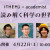Research Fields
Conformal Field Theory, Representation Theory
Term and History
2022-04-01 - Special Postdoctoral Researcher

## Self-introduction

Quantum field theory is a physical theory that describes a wide range of phenomena from the subatomic world to the cosmic scale, and can be regarded as a fruit of human wisdom. However, quantum field theory has not yet been mathematically formulated. This problem perhaps asks us, "How do we formulate space?"
According to the philosophy of algebraic geometry, "space and algebra" are almost the same thing. Here, the algebra is a collection of functions (classical fields). So let us consider the algebra consisting of quantum fields. The "classical algebra" is associative, whereas the "quantum algebra" is non-associative. What should be the corresponding space?

I am currently studying this algebra of quantum fields in the case of two-dimensional quantum field theory. In my previous research, I have worked mathematically on (1) a formulation of the algebra of two-dimensional conformal field theory, (2) constructions of examples, and (3) a construction of non-perturbative deformations. More recently, I have returned to the question of "space" a bit, studying the axioms (consistency) of quantum field theory from a viewpoint of an operad structure of moduli spaces and higher categories.

I was originally a pure mathematician studying representation theory. Crossing the field into physics has been fruitful for my life, although there is still a lot I don't understand. I am very happy to be here at iTHEMS and hope to have good interactions and co-evolution with many researchers.

## Related Events### iTHEMS x academist Online Event "Mathematical Science World 2023"

April 22 (Sat) at 10:00 - 16:30, 2023 Special Lecture### A mathematical formulation of two-dimensional conformal field theory

May 23 (Mon) at 14:00 - 16:30, 2022 Seminar iTHEMS Math Seminar